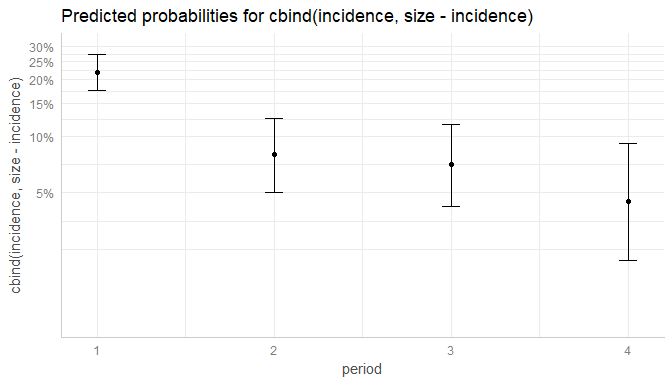# plot()-method

This vignettes demonstrates the plot()-method of the ggeffects-package. It is recommended to read the general introduction first, if you haven’t done this yet.

If you don’t want to write your own ggplot-code, ggeffects has a plot()-method with some convenient defaults, which allows quickly creating ggplot-objects. plot() has only a few arguments to keep this function small and simple. For instance, ci allows you to show or hide confidence bands (or error bars, for discrete variables), facets allows you to create facets even for just one grouping variable, or colors allows you to quickly choose from some color-palettes, including black & white colored plots. Use rawdata to add the raw data points to the plot.

library(ggeffects)
library(sjmisc)
data(efc)
efc$c172code <- to_label(efc$c172code)
fit <- lm(barthtot ~ c12hour + neg_c_7 + c161sex + c172code, data = efc)

# facet by group
dat <- ggpredict(fit, terms = c("c12hour", "c172code"))
plot(dat, facet = TRUE)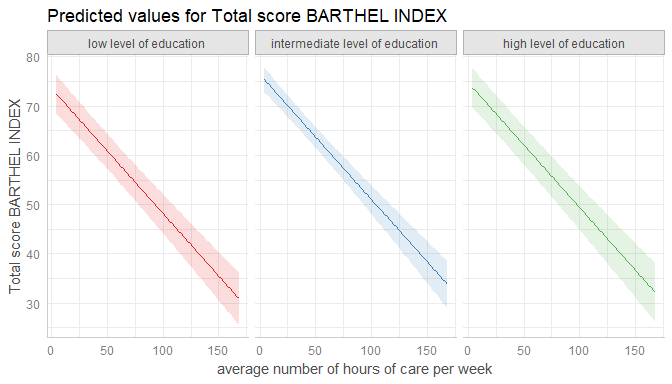# don't use facets, b/w figure, w/o confidence bands
plot(dat, colors = "bw", ci = FALSE)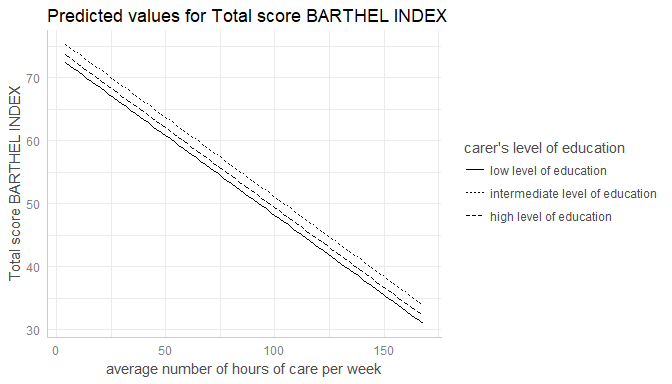# plot raw data
dat <- ggpredict(fit, terms = c("c12hour", "c172code"))
plot(dat, rawdata = TRUE)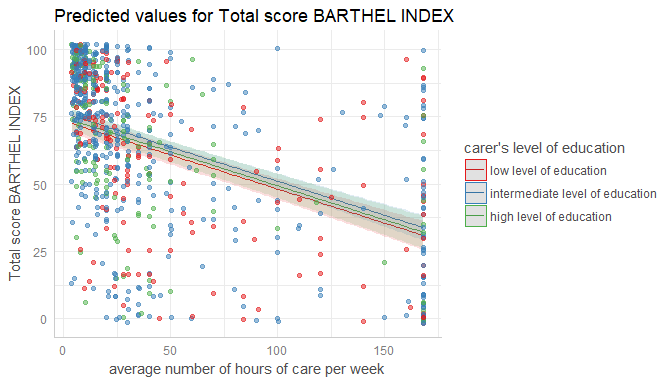# for three variables, automatic facetting
dat <- ggpredict(fit, terms = c("c12hour", "c172code", "c161sex"))
plot(dat)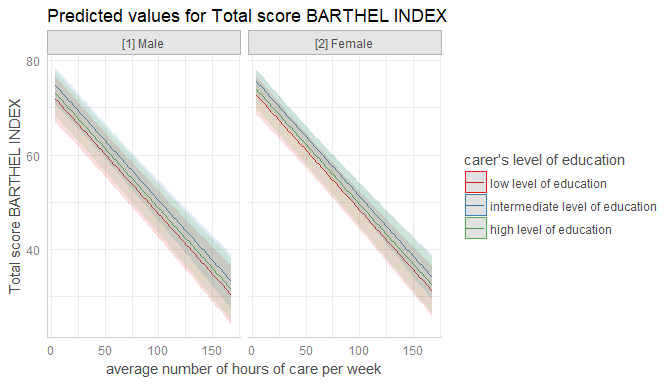# categorical variables have errorbars
dat <- ggpredict(fit, terms = c("c172code", "c161sex"))
plot(dat)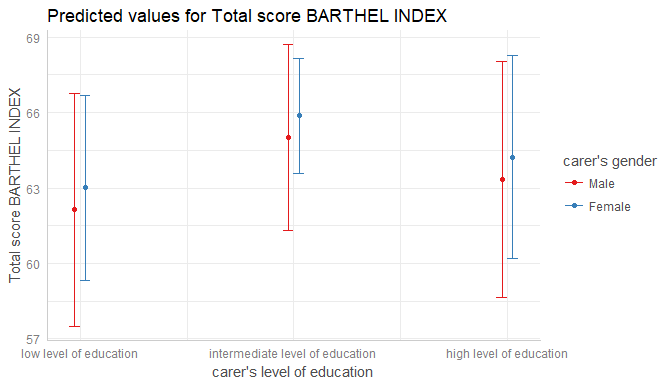# Log-transform y-axis for binomial models

For binomial models, the y-axis indicates the predicted probabilities of an event. In this case, error bars are not symmetrical.

if (requireNamespace("lme4")) {
m <- glm(
cbind(incidence, size - incidence) ~ period,
family = binomial,
data = lme4::cbpp
)

dat <- ggpredict(m, "period")

# normal plot, asymmetrical error bars
plot(dat)
}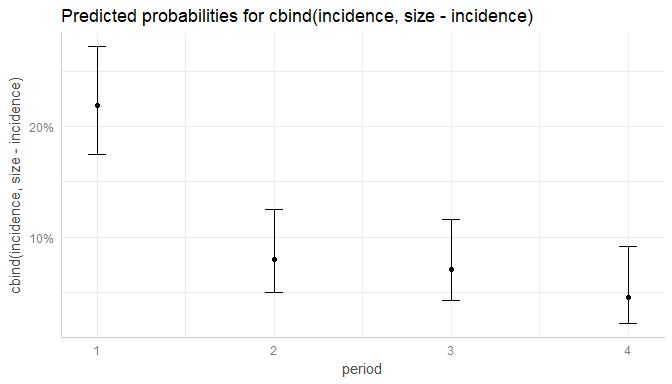Here you can use log.y to log-transform the y-axis. The plot()-method will automatically choose axis breaks and limits that fit well to the value range and log-scale.

if (requireNamespace("lme4")) {
# plot with log-transformed y-axis
plot(dat, log.y = TRUE)
}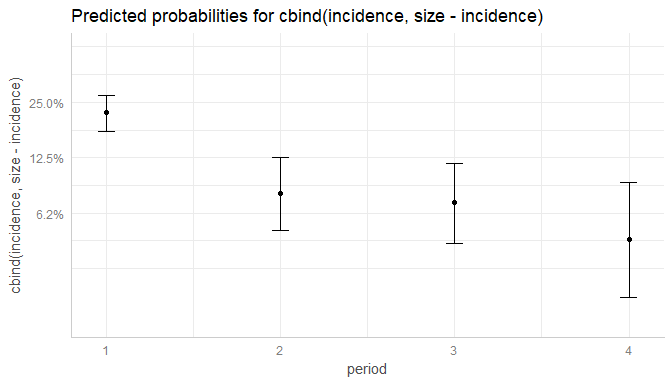# Control y-axis appearance

Furthermore, arguments in ... are passed down to ggplot::scale_y_continuous() (resp. ggplot::scale_y_log10(), if log.y = TRUE), so you can control the appearance of the y-axis.

if (requireNamespace("lme4")) {
# plot with log-transformed y-axis, modify breaks
plot(
dat, log.y = TRUE,
breaks = c(.05, .1, .15, .2, .25, .3),
limits = c(.01, .3)
)
}